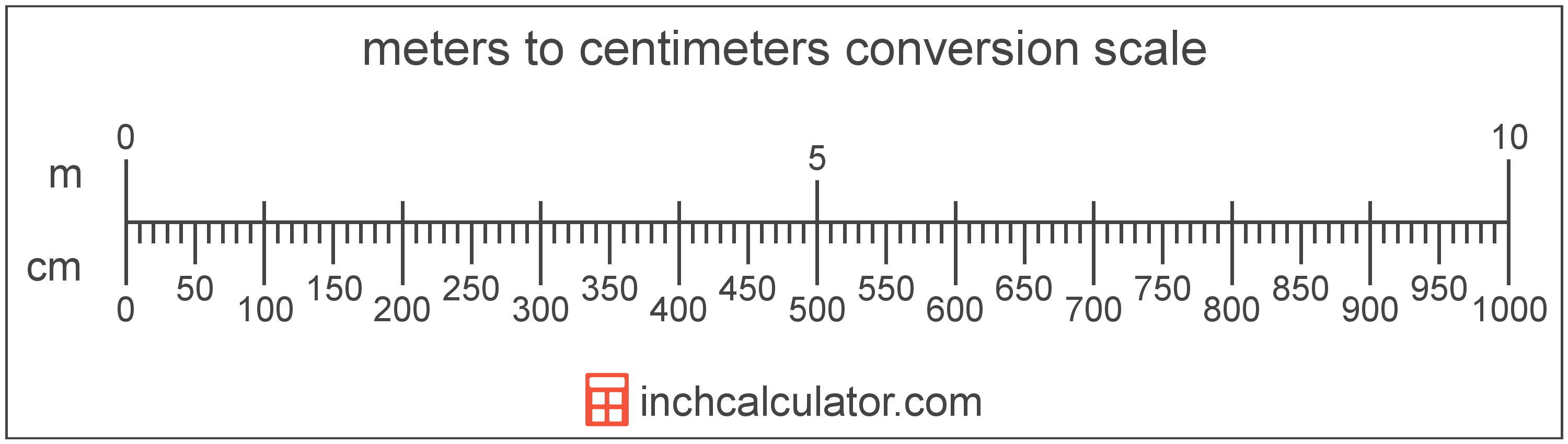# Meters to Centimeters Conversion

Enter the length in meters below to get the value converted to centimeters.

Results in Centimeters:1 m = 100 cm

## How to Convert Meters to CentimetersTo convert a meter measurement to a centimeter measurement, multiply the length by the conversion ratio.

Since one meter is equal to 100 centimeters, you can use this simple formula to convert:

centimeters = meters × 100

The length in centimeters is equal to the meters multiplied by 100.

For example, here's how to convert 5 meters to centimeters using the formula above.
5 m = (5 × 100) = 500 cm

### How Many Centimeters are in a Meter?

There are 100 centimeters in a meter, which is why we use this value in the formula above.

1 m = 100 cm

Our inch fraction calculator can add meters and centimeters together, and it also automatically converts the results to US customary, imperial, and SI metric values.

## Meters

The meter is defined as the length of the path traveled by light in vacuum during a time interval with a duration of 1/299,792,458 of a second, according to the most recent 2019 definition. One meter is equal to 100 centimeters or 39.37 inches.

The meter, or metre, is the SI base unit for length in the metric system. Meters can be abbreviated as m; for example, 1 meter can be written as 1 m.

## Centimeters

One centimeter is equal to one-hundredth (1/100) of a meter, which is defined as the distance light travels in a vacuum in a 1/299,792,458 second time interval.

The centimeter, or centimetre, is a multiple of the meter, which is the SI base unit for length. In the metric system, "centi" is the prefix for 10-2. Centimeters can be abbreviated as cm; for example, 1 centimeter can be written as 1 cm.

Metric rulers typically have 30 cm, which are represented by 30 large tick marks. To get a rough idea of the actual length of a centimeter, a standard pencil is just about 1 cm thick.

We recommend using a ruler or tape measure for measuring length, which can be found at a local retailer or home center. Rulers are available in imperial, metric, or combination with both values, so make sure you get the correct type for your needs.

Need a ruler? Try our free downloadable and printable rulers, which include both imperial and metric measurements.

## Meter to Centimeter Conversion Table

Meter measurements converted to centimeters
Meters Centimeters
0.01 m 1 cm
0.02 m 2 cm
0.03 m 3 cm
0.04 m 4 cm
0.05 m 5 cm
0.06 m 6 cm
0.07 m 7 cm
0.08 m 8 cm
0.09 m 9 cm
0.1 m 10 cm
0.2 m 20 cm
0.3 m 30 cm
0.4 m 40 cm
0.5 m 50 cm
0.6 m 60 cm
0.7 m 70 cm
0.8 m 80 cm
0.9 m 90 cm
1 m 100 cm

## References

1. International Bureau of Weights and Measures, The International System of Units, 9th Edition, 2019, https://www.bipm.org/documents/20126/41483022/SI-Brochure-9-EN.pdf